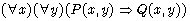4.6.1 When There Are Two Variables

When there are two variables like the following we write two variables.

for x,y st P[x,y] holds Q[x,y]
proof
let x,y;
assume P[x,y];
······
······
thus Q[x,y]; (thesis)
end;

Directly, it means that we demonstrated. assume ..... becomes one logical formula.